## Abstract

The paper examines the primary drivers and factors influencing the volatility of natural gas prices in the world from January 2007 to July 2020. In addition to the narrow dependence between crude oil and natural gas prices, the influence of renewable energy production and coal production on the price of natural gas has been studied. For that purpose, the method of multiple linear regression was used. The results show that the volatility of natural gas prices significantly depends on the type of the shock in the natural gas market, and that the total production of energy from renewable sources, production of coal and natural gas and the price of crude oil have a significant impact on the price of gas.

Keywords: Energy prices, natural gas, multiple linear regressions, renewable energy, price volatility, energy consumption

## 1. Introduction

Natural gas is a mixture of hydrocarbons and methane and because it mixes easily with air and has a high combustion rate without smoke, soot, and solid residues, it has a significant environmental advantage over conventional fuels such as oil and coal . In the same way that oil replaced coal in industrial production at the beginning of the twentieth century, gas suppressed oil at the beginning of the twenty-first century and became an increasingly common fuel used to start the industry. Due to a fact that natural gas is a much cleaner energy source, this tendency in many developed countries is regulated by law, so some industrial sectors are forced to start their production with the help of natural gas. Given the world’s growing concern about the effects of carbon dioxide emissions from fossil fuels, and the impact on climate change and low carbon content in natural gas compared to other fossil fuels, natural gas has become a major energy source in many economies across the globe. Recent research shows that the consumption of natural gas plays an important role in facilitating economic growth in the both short and long term  and that disruptions in the supply of natural gas entail huge economic costs [3-5]. Fluctuations in energy prices are also becoming increasingly important in influencing risk reduction and strategic investments .

The largest recorded shock demand in the history of global natural gas markets happened in the first half of 2020. Faced with historically mild temperatures during the first months of the year, the COVID-19 pandemic hit the already declining demand for gas. Gas consumption is expected to fall by 4% in 2020 [7-10], due to successive lower demand for warm winter heating , the implementation of lockdown measures in almost all countries and territories, to slow the spread of the virus and lower activity generated by the macroeconomic crisis caused by the pandemic. Faced with this unprecedented shock, natural gas markets are undergoing strong supply and demand adjustments, resulting in historically low spot prices and high volatility. Demand for natural gas is expected to gradually recover in 2021, but the COVID-19 crisis will have a longer-term impact on natural gas markets [12-14], as major medium-term drivers are subject to great uncertainty.

With the above mentioned, the contribution of our work to literature is that by using multiple linear regression (MLR) [15,16] it can be proved that selected independent variables are important and necessary in determining the dependent variable and that there is a significant relationship and interaction between selected factors and natural gas prices. It can be also proved that fluctuations in natural gas prices are correlated with certain global economic events. This article also shows how the two most significant depressions in recent history - the global economic crisis and COVID-19, have affected the price of natural gas, which can be useful in further research, forecasting prices and consumption in the energy markets, especially when it comes to increased risk of potentially new infectious diseases in the global market soon.

This paper examines the primary drivers and factors influencing the volatility of natural gas prices in the world from January 2007 to July 2020. In addition to the narrow dependence between crude oil and natural gas prices, the influence of renewable energy production and coal production on the price of natural gas has been studied. For that purpose, the method of multiple linear regression was used, as follows.

## 2. Estimation of natural gas prices

### 2.1 Literature review

Much of the literature on the natural gas market is devoted to examining the relationship between natural gas prices and the crude oil market, suggesting that oil price fluctuations are important determinants of natural gas prices [17-24]. Only a few studies examined the role of supply and demand factors in determining prices in the U.S. natural gas market [25-28]. Some recent studies show that, unlike globally defined crude oil markets, natural gas markets are regionally segmented, suggesting that natural gas prices are formed by regional supply-demand relationships . Following the recent decoupling of the relationship between the crude oil and natural gas markets , it is crucial to understand how natural gas prices respond to supply and demand in times of natural gas market shocks, and to identify the relative contribution factors of each. Accordingly, Nick and Thoenes  use a structural VAR model to examine price volatility in the German natural gas market. They conclude that supply shocks have a significant impact on the German natural gas market and that temperature, storage and supply shocks lead to relatively short-term effects, while oil and coal price shocks result in more lasting effects on gas prices. The relationship between the prices of natural gas and coal was also investigated by Everts et al. . The study used a basic market price replication model with an analysis of the impact of changes on the individual price drivers such as coal prices or subsidies for renewable energy production. The study showed the fall in electricity prices in Germany in the period 2008-2014 can be attributed to market effects such as a reduction in coal use and gas prices as well as a reduction in electricity demand (50%). Only approximately 30% of price reductions could be directly related to subsidies for energy from renewable sources such as wind and solar energy. Mosquera-López and Nursimulu  came to qualitatively similar conclusions. They found that in the short- and medium-term, the main drivers of prices differ and vary in time. In the case of the spot market, determinants of prices were renewable sources and demand for electricity, while in the future markets the main drivers of natural gas prices were the supply and price of coal. Mu  identifies demand for natural gas concerning weather conditions as an important factor influencing the movement of gas prices. Hulshof et al.  conclude that natural gas prices are mainly determined by the weather conditions and availability of stored quantities, while supply has no significant effect. The availability of natural gas in storage and the outside temperature negatively affected the price of natural gas. They also concluded that the price of natural gas was related to the production of electricity from wind.

The outbreak of the COVID-19 pandemic has shaken global financial markets, commodity markets, economic activities, employment, and GDP, in almost all countries of the world. Only a few studies have analyzed the effect of this global pandemic on natural gas prices [36-38]. For this reason, there is a need to study the impact of this phenomenon on the volatility of natural gas prices.

### 2.2 Influencing factors

It is impossible to talk about a single price of natural gas, considering the division of markets in which this energy source is sold. Unlike crude oil, which is traded on stock exchanges and whose prices are uniform worldwide, natural gas prices vary by region. The difference in the price of natural gas is due to different costs of production and transportation, as well as different degrees of liberalization of national natural gas markets. The price of natural gas can be discussed at different levels of the supply chain. The final price of gas at the point of consumption is the sum of the price of production, the price of transportation and the price of local distribution. The price also varies depending on the final consumer, so the industrial sector will usually have a lower price for gas than households do. Thus, in 2007, the average price of natural gas at the level of the European Union was 36% higher for the household sector compared to the industrial sector [39,40]. Another unavoidable factor that contributes to different levels of natural gas prices are taxes that vary from country to country [41,42].

The price of natural gas is experiencing a constant escalation in the market, especially during 2009 because of the global financial crisis of 2008, when the price of natural gas in September was US$2.99 per 1,05 million kJ. This was followed by market recovery and relative price stability during 2010 and subsequent years [7-10]). However, natural gas prices fell sharply since the beginning of 2020 due to weak demand and abundant supply. European natural gas prices fell to a decade-long low and have fallen by almost 40% since early 2020 [39,40]. U.S. natural gas prices have fallen slightly, in part because they had already been low [25-28]. Price differences between regional benchmarks for natural gas continued to decline, mostly driven by the increasing availability of liquefied natural gas. However, the fall in prices of natural gas and coal is smaller than the price of crude oil, which has fallen by about 65% since January 2020. Natural gas and coal are primarily used for electricity generation and industrial purposes, not for transportation. As such, lockdowns and travel restrictions had less of an impact on demand for these products than for crude oil . The onset of the COVID-19 pandemic created an unprecedented shock to the global energy system, and gas consumption around the world was significantly affected. Demand for natural gas has fallen in the electricity sector due to lower electricity usage, and in the industrial and commercial sectors due to the shutdown of factories and businesses. The demand for gas among the population did not decrease while people stayed at home. These impacts varied by region. In Europe, gas demand fell by 7% year-on-year during the first five months of 2020 [7-10]. In the first quarter, the decline was driven by mild winters and higher production from renewable sources. However, lockdown measures began to affect gas demand from March on, where May consumption was already 11% lower than in March [7-10]. Natural gas and coal have seen significant reductions in demand, as lockdown measures have reduced industrial activity and electricity consumption, while extremely warm winters have also reduced demand in early 2020. The International Energy Agency estimates that lockdown measures have reduced electricity demand to at least 15% in several major economies, including France, Italy, Spain, and the United Kingdom [7-10]. In the United States, the U.S. Energy Information Administration expects U.S. demand for electricity to fall by about 5%, and a moderate decline in housing use will offset a greater decline in commercial and industrial use - sectors affected by lockdowns [7-10]. Natural gas prices are expected to recover from the current low but will still be about 25% lower than in 2019 [7-10]. Demand for natural gas is expected to gradually recover from current low levels after lifting the lockdown but will remain below 2019 levels. Demand for coal is likely to remain weaker, as a part of the ongoing transition from coal to natural gas in global electricity generation. Global gas demand could fall by 4% annually in 2020 [7-10]. This would be the largest drop in gas demand ever recorded since the development of the gas industry in the second half of the 20th century. By comparison, gas demand fell by 2% in 2009 due to the global financial crisis. About 75% of demand loss is likely to occur in developed gas markets across Europe, North America, the Commonwealth of Independent States (CIS), and Asia due to lower electricity demand, declining industrial activity, and lower space heating needs in the commercial sector. Production and consumption of natural gas from January 2007 - September 2020 are given in Figure 1, production of coal and energy from renewable sources from January 2007 - September 2020 in Figure 2, while prices of crude oil and natural gas from January 2007 - September 2020 in Figure 3.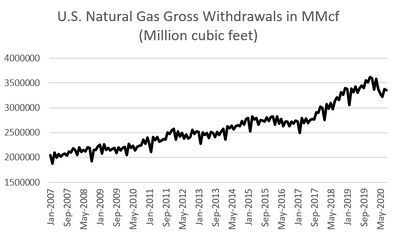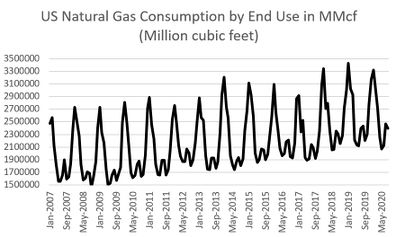Figure 1. Fluctuation of production and consumption of natural gas from January 2007-September 2020. Source: U.S. Energy Information Administration - Monthly Energy Review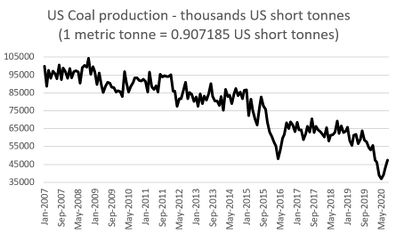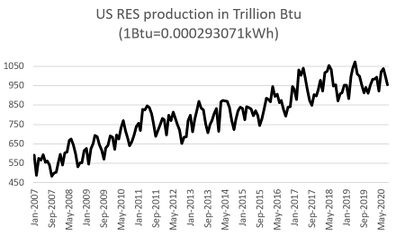Figure 2. Fluctuation of production of coal and energy from renewable sources from January 2007-September 2020. Source: U.S. Energy Information Administration - Monthly Energy Review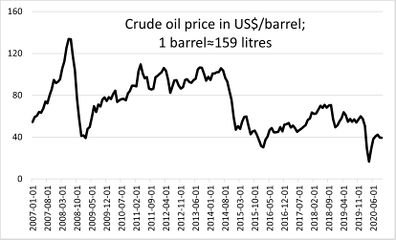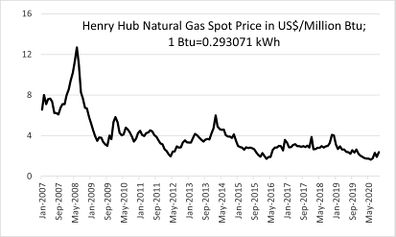Figure 3. Fluctuation of prices of crude oil and natural gas from January 2007-September 2020. Source: FRED Graph Observations, and Henry Hub Natural Gas Spot Price, respectively As we can see in Figure 3, the market shock caused by the global economic crisis led to a fall in the price of natural gas from 12.69 US$ per 1.05 million kJ of June 2008 to 2.99 US$in September 2009. Also, the pandemic COVID -19 caused a drop in price from 4.09 US$ (December 2019) to 1.63 US$per 1.05 million kJ as the price was in June 2020. ### 2.3 Methodology. Multiple linear regression To identify the dependence of natural gas prices on different shocks in the natural gas market, monthly time intervals were used during the period from January 2007 to September 2020. This period was used to cover two major shocks in the natural gas market: the 2008 global economic crisis and the COVID-19 virus pandemic. Monthly data have sufficient frequency to analyze the effects of price dependence and market spillovers over time . As a source of data for total production and total consumption of natural gas in billions of cubic feet, a database published by the EIA (U.S Energy Information Administration) was used. The price of crude oil (US$ per barrel) is from the Federal Reserve Economic Data - FRED, and for the actual price of natural gas (US\$ per 1.05 million kJ) the data published by Henry Hub Natural Gas Spot Price was used. Data on coal production (in thousands of tons) as well as data on energy production from renewable energy sources (RES) in 1.05 million kJ were taken from the EIA.

The Multiple Linear Regression analysis is conducted using a mathematical expression of this model  as follows:

 ${y}_{i\,}=\,{\beta }_{0\,}+\,{\beta }_{1}{x}_{1i\,}+\,\cdots \,+\,{\beta }_{k}{x}_{ki}\,+{\varepsilon }_{i}$
(1)

where $y$ is the dependent variable, ${\beta }_{0}$ the regression constant, $x_{1}$, $x_{2}\cdots x_{k}$ the independent variables, $\varepsilon$ the standard error i.e., residues and $\beta$ the unknown parameter to be evaluated (regression parameter).

Values of the regression parameters with each independent variable show how much the dependent variable changes on average when the observed independent variable increases by one of its units, and all other independent variables remain unchanged.

In this model, all assumptions apply as in the simple regression model with two additional assumptions, so that the parameter estimates have their desired properties:

• the sample size must be greater than the number of evaluation parameters,
• regressors should not be perfectly correlated with each other (linearly dependent).

Sample estimates of unknown parameters are obtained by applying the method of least squares. In the case of models with two independent variables, the following function needs to be minimized:

 $F\left({\hat {b}}_{0},{\hat {b}}_{1},\cdots ,{\hat {b}}_{k}\right)=\sum _{i=1}^{n}{\epsilon }_{i}^{2}=\sum _{i=1}^{n}{\left({y}_{i}-{\hat {y}}_{i}\right)}^{2}=\sum _{i=1}^{n}{\left({\hat {b}}_{0}+{\hat {b}}_{1}\cdot {x}_{1i}+{\hat {b}}_{k}\cdot {x}_{2i}\right)}^{2}$
(2)

where $F$ is the function to be minimized, ${\textstyle {\hat {b}}_{0},{\hat {b}}_{1},\cdots ,{\hat {b}}_{k}}$ the estimates of the corresponding coefficients of their standard error (roots of the estimates of their dispersions), ${\textstyle \epsilon }$ the standard error i.e., residues, ${\textstyle {y}_{i}-}$${\hat {y}}_{i}$ the difference between the actual and the predicted value of the dependent variable and $n$ the number of variables.

Minimization of the function $F$ is achieved by equalizing its partial derivatives of the first order by parameters, with zero:

 ${\begin{matrix}\displaystyle {\frac {\partial F}{\partial {\hat {b}}_{0}}}=0\\\displaystyle {\frac {\partial F}{\partial {\hat {b}}_{1}}}=0\\{\begin{matrix}\vdots \\\displaystyle {\frac {\partial F}{\partial {\hat {b}}_{k}}}=0\end{matrix}}\end{matrix}}$
(3)

By arranging these equations, a system of normal equations is obtained:

 $\sum _{i=1}^{n}{y}_{i}=n\cdot {\hat {b}}_{0}+{\hat {b}}_{1}\cdot \sum _{i=1}^{n}{x}_{1i}+\ldots +{\hat {b}}_{k}\cdot \sum _{i=1}^{n}{x}_{ki}$
(4)
 $\sum _{i=1}^{n}{{x}_{1i}\cdot y}_{i}={\hat {b}}_{0}\cdot \sum _{i=1}^{n}{x}_{1i}+{\hat {b}}_{1}\cdot \sum _{i=1}^{n}{x}_{1i}^{2}\ldots +{\hat {b}}_{k}\cdot \sum _{i=1}^{n}{{x}_{1i}\cdot x}_{ki}$
(5)

whose solution yields sample grades ${b}_{0}$, ${b}_{1},\cdots ,b_{k}$, of unknown parameters $\beta _{0},\cdots ,\beta _{k}$.

The equations of the observed system can be presented in matrix notation:

 $X'\cdot Y=\left(X'\cdot X\right){\hat {b}}$
(6)

where $X'$ is transposed matrix of matrix $X$, follows:

 ${X}^{'}\cdot Y=\left[{\begin{matrix}\displaystyle \sum _{i=1}^{n}{Y}_{i}\\\displaystyle \sum _{i=1}^{n}{x}_{1i}\cdot {Y}_{i}\\{\begin{matrix}\vdots \\\displaystyle \sum _{i=1}^{n}{x}_{ki}\cdot {Y}_{i}\end{matrix}}\end{matrix}}\right]$
(7)
 ${X}^{'}\cdot X=\left[{\begin{matrix}n&\displaystyle \sum _{i=1}^{n}{x}_{1i}&{\begin{matrix}\ldots &\displaystyle \sum _{i=1}^{n}{x}_{ki}\end{matrix}}\\\displaystyle \sum _{i=1}^{n}{x}_{1i}&\displaystyle \sum _{i=1}^{n}{x}_{1i}^{2}&{\begin{matrix}\ldots &\displaystyle \sum _{i=1}^{n}{x}_{1i}\cdot {x}_{ki}\end{matrix}}\\{\begin{matrix}\vdots \\\displaystyle \sum _{i=1}^{n}{x}_{ki}\end{matrix}}&{\begin{matrix}\vdots \\\displaystyle \sum _{i=1}^{n}{x}_{1i}\cdot {x}_{ki}\end{matrix}}&{\begin{matrix}{\begin{matrix}\ddots \\\ldots \end{matrix}}&{\begin{matrix}\vdots \\\displaystyle \sum _{i=1}^{n}{x}_{ki}^{2}\end{matrix}}\end{matrix}}\end{matrix}}\right]$
(8)
 ${\hat {b}}=\left[{\begin{matrix}{\hat {b}}_{0}\\{\hat {b}}_{1}\\{\begin{matrix}\vdots \\{\hat {b}}_{k}\end{matrix}}\end{matrix}}\right]$
(9)

By solving the matrix equation, a solution for the evaluation vector is obtained ${\textstyle {\hat {b}}}$:

 ${\hat {b}}={\left(X'\cdot X\right)}^{-1}\left(X'\cdot Y\right)$
(10)

To test the significance of the estimated regression parameters, it is necessary to know their distributions. In case the model assumptions are met, these parameters have the following distribution:

 ${\hat {b}}:N\left(\beta ;{\sigma }^{2}{\left(X'X\right)}^{-1}\right)$
(11)

where $N$ is the number of parameters, $\beta$ is the vector of regression population parameters and ${\textstyle \sigma }$ is the accidental error. In that case, testing the significance of the mentioned parameters can be performed using the following distribution:

 $T=\displaystyle {\frac {\hat {{b}_{j}}}{\sqrt {\frac {{a}_{ii}\cdot \sum _{i=1}^{n}{\epsilon }_{i}^{2}}{n-k-1}}}}}$
(12)

This random variable, in the case of the correct hypothesis $H_{0}$ (null hypothesis - which assumes that there is no significant relationship between the dependent and the independent variable), $\beta _{j}$, has a $T$ distribution with $n-k-1$ degrees of freedom, where the $k$ is the number of independent variables, аnd $a_{ii}$ is $i$-th diagonal element of the matrix $(X'X)^{-1}$.

The basic assumption in the formation of a multilinear regression model is the normal distribution of residual variable models. Table 1 shows the minimum and the maximum values of all variables and their standard deviations (Standard Deviation) between the minimum and the maximum values in the observed period.

Table 1. Original variables with parameters from descriptive statistics
Variable Minimum Maximum Mean Std. deviation
Natural Gas Price 1.63 12.69 3.95 1.96
Crude Oil Price 16.55 133.88 71.73 23.73
Natural Gas Production 1878.00 3.620.71 2.603,39 426.78
Natural Gas Consumption 1460.04 3.417.20 2192.16 450.96
Renew. Eng. Source Prod. - RES 483.32 1063.77 789.28 142.78
Coal Production 36934.07 104389.50 77800.98 15804.46

SPSS (Statistical Package for the Social Sciences) version 23 and XLStat (Statistical Software for Excel) premium software are used to build regression models.

To assess the quality of the acquired regression models, i.e., to assess the reliability and the impartiality, several econometric tests need to be performed:

1. Absence of multicollinearity. This test uses two determinants: Variance Inflation Factor (VIF) and Tolerance Factor (TOL). If the tolerance value is less than 0.2 or 0.1 and at the same time the VIF value is 10 or more, then multicollinearity is problematic. The problem of multicollinearity does not exist when the values of VIF are <5 and 10, respectively, while the values of TOL are <0.2 and 0.1, respectively. Variables whose VIF values are greater than 10 and TOL> 0.4 indicate a multicollinearity problem and should not be in the model.
2. Normal distribution of variables in the model. It is tested using Shapiro-Wilk and values of two coefficients: asymmetry (Skewness) and flatness (Kurtosis) for which acceptable values of variables are approximately ± 1.
3. Control of the random error of homoskedasticity or heteroskedasticity. Random errors should show the same level of distribution around their mean value. When random variances of errors differ significantly from each other, it is a case of heteroskedasticity. This testing will be performed by Breusch-Pagan (based on testing the dependence of residual variation on the height of all regressors) and White's test (based on comparing the variance of estimators obtained by the method of least squares under conditions of homoskedasticity and heteroskedasticity).
4. Autocorrelation of residues is a common occurrence in the case of economic time series. The Durbin-Watson test is used to detect autocorrelation. Values of this test can range from 0 to 4. Values greater than 2 indicate negative, while values less than 2 indicate positive autocorrelation.

Results of the Pearson correlation coefficient (test statistics that measures the statistical relationship between two continuous variables), show a relatively strong statistical relationship between the price of natural gas and the price of crude oil, natural gas production, renewable energy production and coal production, except for gas consumption where the natural gas price relationship is weak. The highest level of positive correlation with the dependent variable is achieved by coal production (0.654), followed by the price of crude oil (0.527), while the gas production (-0.629) and energy production from renewable sources (-0.663) variables are highly correlated, but with the opposite sign. The correlation matrix is given in Table 2.

Table 2. Pearson correlation matrix
Variable (1) (2) (3) (4) (5) (6)
(1) 1.000 0.527 -0.629 -0.264 -0.663 0.654
(2) 0.527 1.000 -0.490 -0.284 -0.404 0.635
(3) -0.629 -0.490 1.000 0.425 0.865 -0.848
(4) -0.264 -0.284 0.425 1.000 0.406 -0.447
(5) -0.663 -0.404 0.865 0.406 1.000 -0.843
(6) 0.654 0.635 -0.848 -0.447 -0.843 1.000
(1) Natural Gas Price. (2) Crude Oil Price. (3) Natural Gas Production. (4) Natural Gas Consumption.
(5) Renewable Energy Source Production - RES. (6) Coal Production

Table 3 shows the Skewness and Kurtosis coefficients. Results for all variables are within the acceptable value of ± 1. The value of the Shapiro -Wilk test is also within the acceptable limit values, so it can be concluded that a normal distribution of variables in the model has been achieved.

Table 3. Checking the normality of the distribution of dependent variables in the model
Variables Skewness Kurtosis
Statistic Std. Error Statistic Std. Error
Crude Oil Price 0.208 0.190 -0.739 0.377
Natural Gas Production 0.655 0.190 -0.394 0.377
Natural Gas Consumption 0.636 0.190 -0.392 0.377
Ren. Eng. Source Prod. - RES -0.128 0.190 -0.781 0.377
Coal Production -0.515 0.190 -0.694 0.377
Shapiro-Wilk: 0,961

Results of the regression model are given in Table 4. The value of the coefficient of the multiple correlation (R) serves to determine the quality of the prediction for the independent variable, in this case, Natural gas price. The value of 0.723 represents a good prediction. The value of the multiple determination coefficient (R Square) is relatively high and states that the model explains 52.3% of the dependent variable. The value of the standard error of the estimate (Std. Error of the Estimate) indicates that the set model is accurate, while the Durbin-Watson test indicates a positive autocorrelation (the result is less than 2), which is a good sign for our regression model.

Table 4. Multiple correlation, determination, and autocorrelation coefficients
R R Square Adjusted R Square Std. error of the Estimate Change Statistics Durbin-Watson
R Square Change F Change
0.723 0.523 0.507 1.37743 0.523 34.586 0.238
Model Summary. Predictors: (Constant), Coal Production, Natural Gas Consumption, Oil Price, Renewable Energy Sources
Production (RES), Natural Gas Production; Dependent Variable: Natural Gas Price

The ANOVA (analysis of variance) method was used to test the null hypothesis on the coefficient of multiple determinations. ANOVA is a test of the hypothesis that is appropriate to compare means of a continuous variable in two or more independent comparison groups. The results indicate that the null hypothesis can be rejected, i.e., it makes sense to use this model to explain the dependent variable (Table 5).

Table 5. Estimation of the significance of partial regression coefficients
Model Sum of Squares $D_{f}$ Mean Square F Sig.
Regression 328.105 5 65.621 34.586 0.000
Residual 299.776 159 1.897
Total 627.881 164

The significance of partial regression coefficients was determined by performing a T-test. From Table 6 it is possible to conclude based on coefficients in the column Sig. to make a unique contribution to the model, i.e., that the statistically significant variables “Crude Oil price” and “RES Production” (the same conclusion can be reached on basis of 95% confidence interval, which only in the case of the mentioned variables does not include 0). As values of the inflation factor of variance - VIF and the tolerance factor - Tol., are within acceptable limits, it can be concluded that all variables can be part of the regression model, so the value of the independent variable Y is the sum of the values of the coefficient B other than zero.

Table 6. Estimation of the significance of partial regression coefficients
(1) (2) (3) (4) (5) Collinearity Statistics (6) (7)
Lower Bound Upper Bound
(Constant) 7.568 2.541 2.978 0.003 2.549 12.587
Crude oil price 0.025 0.006 0.301 4.001 0.000 0.013 0.037 0.535 1.870
Nat. Gas Production 0.000 0.001 -0.063 -0.515 0.608 -0.001 0.001 0.202 4.957
Nat. Gas Consumption 0.000 0.000 0.057 0.920 0.359 0.000 0.001 0.793 1.262
Renewable Energy Sources Production - RES -0.007 0.002 -0.495 -3.930 0.000 -0.010 -0.003 0.191 5.246
Coal Production 0.000 0.000 0.018 0.130 0.897 0.000 0.000 0.162 6.168
(1) Unstandardized Coefficients B. (2) Stand. Coefficients - Std. Error. (3) t – Beta. (4) Sig. (5) 95.0% Confidence Interval for B. (6) Tol. (7) VIF

Based on Table 6, the natural gas price can be estimated using Eq. (13):

 Y (Natural Gas Price) =7.568 + 0.025 (Crude Oil Price) – 0.007 (RES Production)
(13)

Based on values in Table 7, it can be concluded that the homogeneity of variances and predictive values deviates from the limit values, so that in this case a pronounced heteroskedasticity exists.

Table 7. Heteroskedasticity tests
Heteroskedasticity Test Breusch-Pagan White
Critical value 3.8414588 5.9914645
Observed value R-squared 16.1229036 20.6487180

As the influence of non-constant variance of errors on the efficiency of estimation by the least square method as well as the validity of its conclusions, depends on several factors including sample size, degree of predictor variation, regressor values and the relationship between error variance and independent variables, it is not possible to reach full general conclusions relating to the consequences produced by heteroskedasticity in this case. Some authors believe that heteroskedasticity is not a big problem in econometric research. One of them is N. Gregori Mankiw who argues that “heteroskedasticity has never been a reason to reject an otherwise good model.” . On the other hand, John Fox  points out that “unequal variances of errors should be corrected only if the problem is serious.” As previous tests have led to the conclusion that the regression model in work is good, it can be believed that there is no need to correct the data by applying possible forms of transformation.

## 3. Conclusions

Natural gas is one of the most used non-renewable hydrocarbons. Like almost any non-renewable energy source, it releases carbon dioxide during combustion. The linear model identified that one of the most important factors influencing the prices of natural gas is green energy or energy obtained from renewable sources (solar energy, wind energy, etc.). Namely, the presence of this factor in the model is statistically significant with a high correlation coefficient with the price of natural gas - with a negative sign as the volume of natural gas production. Traditionally, a significant factor is the price of crude oil, which is statistically significant in the model and has a positive high correlation coefficient as well as coal production.

It is difficult to predict the expected volatility of natural gas prices in the future because factors of influence depend on global crises and shocks as well as on the geopolitical situation in the world. Investors in the natural gas market can use models formulated in this way to make investment decisions. Our findings highlight the importance of including pandemic risk in forecasting global gas supply and demand. The results of the research will help policymakers assess the impact of the COVID-19 pandemic on natural gas price volatility and formulate appropriate plans and strategies to reduce the impact and improve forecasting models that should include pandemics, natural and economic disasters.

Our proposed method can be used for the prediction of future natural gas prices, in addition to other techniques such as wavelets, time series, symbolic regression [47-49], artificial neural networks [50,51], cointegration technique , Monte Carlo , etc.

## Acknowledgements

This research has been supported by the Ministry of Education, Science, and Technological Development of the Republic of Serbia. Dejan Brkić acknowledges additional support by the Technology Agency of the Czech Republic through the project CEET—"Center of Energy and Environmental Technologies" TK03020027.

Conflicts of interest: The authors declare no conflict of interest. All authors have approved the final version of this paper.

### Document informationPublished on 15/12/21
Accepted on 02/12/21
Submitted on 12/04/21

Volume 37, Issue 4, 2021
DOI: 10.23967/j.rimni.2021.12.002

### Document Score0

Views 190
Recommendations 0